# Taming Cox-Ross-Rubinstein Trees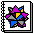Download the notebook

``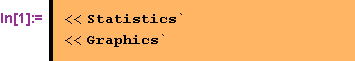``

### Implementing a CRR tree in Mathematica

Implementing Cox/Ross/Rubinstein trees for option valuation is discussed in Shaw (1998). Using the functionality which is available in Version 4 of Mathematica the following very fast implementation for european options is possible (to price american options only two slight modifications are necessary):

````

Using some data to test the result gives the following result.

````
`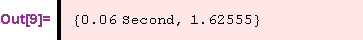`

To get a feeling for the accuracy of this value we implement the Black-Scholes formula.

``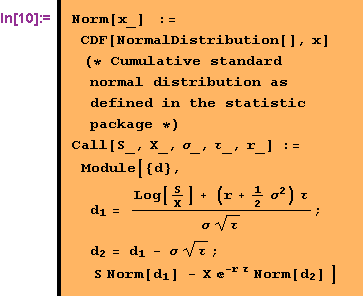``

and calculate the analytical value.

``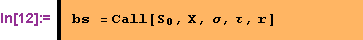``
`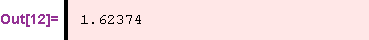`

### Oscillating behavior

It is well known that the binomial methods does not converge perfectly. Instead it shows oscillating behavior (see Omberg (1987)). These oscillations are present even if the number of steps is quite high. Following Shaw (1998) it is quite simple to visulize these oscillations. (Before starting the next input line in Mathematica be aware that there quite a lot of calculation you demand. So allow Mathematica some seconds to finish the calculations.)

````The red line describes the analytical solution.

To reduce the oscillations some trading systems allow to take the mean of stepsandwhen valuing options with binomial trees. valuation methods. This procedure leads to the following picture.

``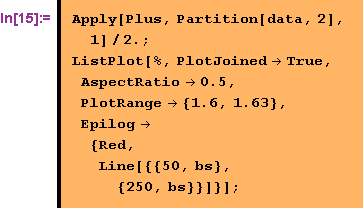``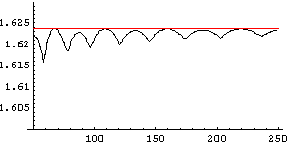As can be seen from the picture taking the mean of the result of successiv number of steps is not yet the solution of the problem.

### Improving the convergence behavior

From a participant of our last training in Zurich we were reminded on an improvement to the tree which reduces the oscillation behavior quite drastic. When instead of the known option prices at maturity of the option the prices calculated with the Black-Scholes formula one step before maturity are taken as the starting value for working backwards through the tree (see the following implementation) the oscillation vanishes almost completely.

``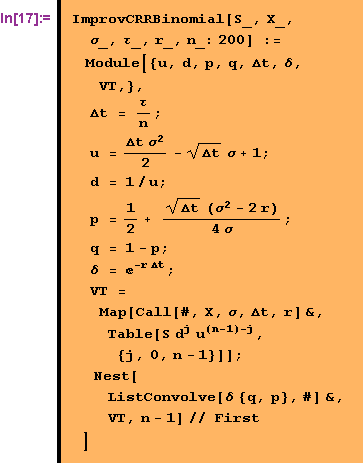``

This adjustment works also for the valuation of american options, since the american and european options are not distinguishable (in a tree sense) one step before maturity.

Now looking at the timing it can be seen that this improvement slows down the tree considerable.

``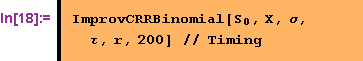``
`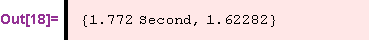`

To speed things up we use a compiled version for the Black-Scholes formula, which cuts the calculation time into half. But it is still considerable higher then the first version.

````
`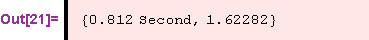`

But looking at the same graphic it can be seen that the improvement in accuarcy is quite impressive (All graphics have the same plot range).

````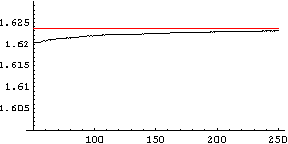### References

Cox, J., Ross, S., Rubenstein, M., 1979, Option Pricing: A Simplified Approach, in: Journal of Financial Economics 7, p. 229-263.

Omberg, E., 1987, A Note on the Convergence of Binomial-Pricing and Combound-Option Models, in: Journal of Finance 42, p. 463-469.

Shaw, W., 1998, Modelling Financial Derivatives with Mathematica, Cambridge University Press.

Converted by Mathematica      January 8, 2001